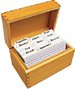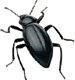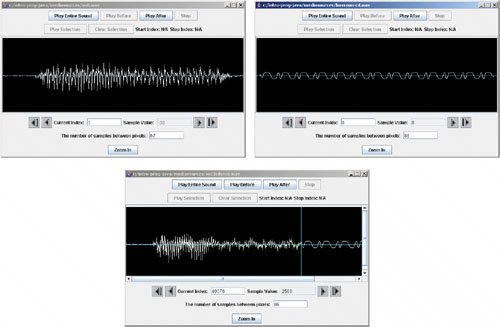# Section 10.2. Blending Sounds

[Page 313 (continued)]

### 10.2. Blending Sounds

In this example, we take two soundssomeone saying "Aah!" and a bassoon instrument sound of C in the fourth octaveand blend the two sounds. The way we do this is to first copy part of the first sound, "Aah!", then copy 50% of each sound, and then copy the rest of the second sound (Figure 10.1). This is very much like mixing 50% of each sound at a mixing board. It's also very much like the way that we blended pictures in Program 28 (page 156)!

[Page 314]

##### Figure 10.1. The top and middle waves are added together to create the bottom wave. (This item is displayed on page 313 in the print version)##### Program 80. Blending Two Sounds`/** * Method to overlap or blend two sounds. Start * by copying the first 20,000 samples from sound1 into * the current sound then copy the sum of half of sound1 * and half of sound2 for the next 20,000 samples and * end with the next 20,000 samples from sound2. */ public void blendSounds() { Sound sound1 = new Sound(FileChooser.getMediaPath("aah.wav")); Sound sound2 = new Sound(FileChooser.getMediaPath("bassoon-c4.wav")); int value = 0; // copy the first 20,000 samples from sound1 into target for (int index=0; index < 20000; index++) this.setSampleValueAt(index, sound1.getSampleValueAt(index)); // copy the next 20,000 samples from sound1 and blend that // with the first 20,000 samples from sound2 for (int index = 0; index < 20000; index++) { value = (int) ((sound1.getSampleValueAt(index + 20000) * 0.5) + (sound2.getSampleValueAt(index) * 0.5)); this.setSampleValueAt(index + 20000,value); } // copy the next 20,000 samples from sound2 into the target for (int index=20000; index < 40000; index++) this.setSampleValueAt(index + 20000, sound2.getSampleValueAt(index)); }`## Common Bug: Set the Media Folder First!

If you're going to write code that uses FileChooser.getMediaPath(String baseName), you'll need to execute FileChooser.setMediaPath(String directory) first.

Like blending the picture (Program 28 (page 156)), there are loops in this method for each segment of the blended sound.

[Page 315]
• We start by creating the sound1 and sound2 sounds for blending. The length of these sounds is over 40,000 samples, but we're just going to use the first 40,000 as an example.

• In the first loop, we simply get 20,000 samples from sound1 and copy them into the current sound this. Notice that we're not using a separate index variable for the targetinstead, we're using the same index variable, index, for both sounds since we are copying from 0 to 19,999 from sound1 into 0 to 19,999 in the current sound.

• In the next loop, we copy 20,000 samples from both sound1 and sound2 blended into the current sound. We get a sample from each of sound1 and sound2, then multiply each by 0.5 and add the results together. The result is a sample that represents 50% of each. Notice that we are using one index variable here as well but adding 20,000 to the value of that for determining the index of sound1 and the current sound. So we blend values from sound1 starting at index 20,000 and from sound2 starting at index 0 and the blended values go into the current sound starting at index 20,000.

• Finally, we copy another 20,000 samples from sound2. The result sounds like "Aah," first, then half of each, then just a bassoon note. Notice that we start the index at 20,000 for the next place to copy from sound2. This means we need to add 20,000 to that value for the index in the current sound (since there are already 40,000 values in the current sound).

To create the blended sound, first create a sound using the file that has 3 seconds of silence. Then explore it to see what it looks like before the blending (Figure 10.2). Next, blend the two sounds into the silent sound. Finally, explore the new sound.

`> String fileName = FileChooser.getMediaPath("sec3silence.wav"); > Sound target = new Sound(fileName); > target.explore(); > target.blendSounds(); > target.explore();`

##### Figure 10.2. The original "ahh" sound, the original bassoon note, and the blended sound. (This item is displayed on page 316 in the print version)Introduction to Computing & Programming Algebra in Java(c) A Multimedia Approach
ISBN: N/A
EAN: N/A
Year: 2007
Pages: 191

flylib.com © 2008-2017.
If you may any questions please contact us: flylib@qtcs.net